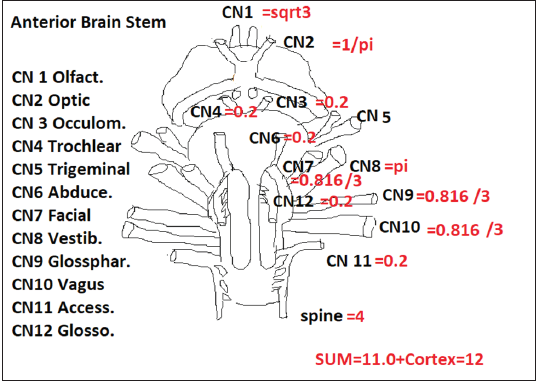• Submissions# Evolution of the Cranial Nerves

Paul TE Cusack*

*Corresponding author: Paul T E Cusack, BScE, DULE, Saint John, Canada

Submission: December 14, 2020;Published: December 21, 2020ISSN: 2640-9666
Volume4 Issue2

#### Abstract

In this brief paper, we consider why there are 12 cranial nerves from an evolutional and mathematical point of view. We see that AT Math once again, plays an important role in determining the evolution of the nervous system.

Keywords: Cranial nerves; Brain stem; Senses; Golden mean parabola

#### Introduction

In the human brain, we have 12 pairs of cranial nerves. We consider here only 10 because the first two, Olfactory and Optic, do not go through the brain stem. The brain stem, of course is made up of three compartments: Midbrain; Pons; and Medulla. The Medulla joins the brain stem to the spinal cord . There are 31 pairs of spinal nerves. Interestingly, 31 is the 12th prime number from mathematics. So, the nervous system can be divided into the sensory neurons and motor neurons. In a previous paper, this author has established that there can be assigned values to the various neurons .

They are as follows:

1. Smell =sqrt3
2. Sight =1/Pi
3. Hearing =Pi
4. Taste=sqrt G=0.816
5. And touch =4
6. Motor=1
These sum to 2.
If we drop smell (olfactory) and sight (optic) as mentioned, then we have:
1. CN7,9,10=Taste=0.816
2. CN8 =Hearing =Pi
3. Touch=Spinal Cord=4
4. Motor=1
These sum to Capacitance =8.95759~ c^2 (Figure 1).
Now, if we consider the basic R_L_C circuit, we have:
V=iR
105=i(0.4233+1/c^2-2)
105=I x 146.543
i=0.717875~72

Figure 1:Brain stem and cranial nerves.Consider:
SE=E x M
=-1/8 x 1/9
=139.08~1.388=1/72
i=t^2
=1/1.3808= t^2~1/72=1/E=t
Now we shall consider the nervous system in entirety.
1. Cerebellum=Motor=1
2. Cerebral Cortex
a. CN 1= Olfactory=sqrt3
b. CN 2= Optic=1/Pi
3. Cranial Nerves
a. CN7,9,10=taste=sqrt G=0.816
b. CN 8=Hearing=Pi
4. Spine =Touch=4
SUM=10.005~1.000
V=iR
R=1/Capacitance=1/10.005=0.1
V=(1/ c^2)(1/10)
=1/89.87~1/9
SE=(ExM)=(-1/8 x 1/9)=1/7.2=1.3888
Power P=i^2R
I= t^2
P=t^4(10.005)
=(0.8479)^4(10)
=516.8
GMP= E=-1.2497~-1.25

#### Conclusion

We see that the cranial nerve evolution respects the laws of physics.

1. Goldberg S (2019) Clinical neuroanatomy made ridiculously simple. Med Master.
2. Diamond MD (1985) The Human Brain Coloring Book., Collins.

We at Crimson Publishing are a group of people with a combined passion for science and research, who wants to bring to the world a unified platform where all scientific know-how is available read more...